# Octal Hexadecimal Number Systems Digital Electronics What More

• Slides: 25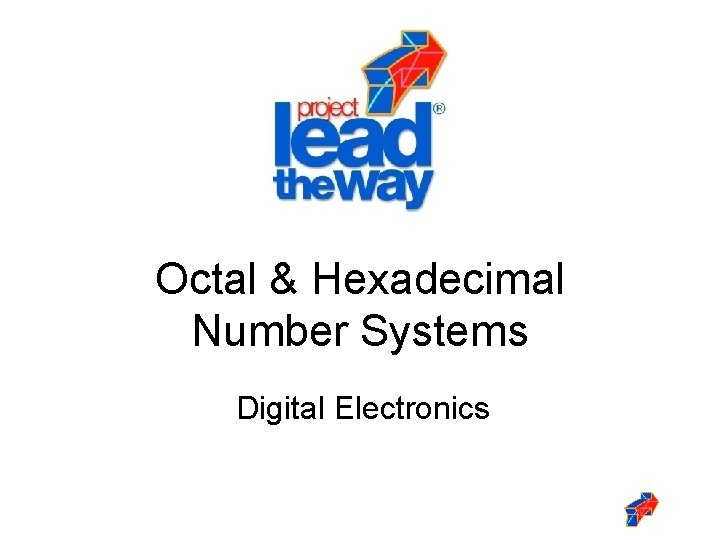Octal & Hexadecimal Number Systems Digital Electronics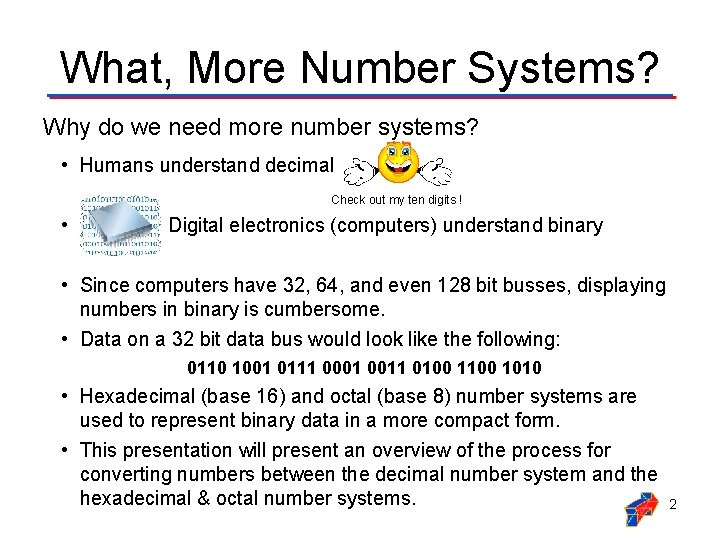What, More Number Systems? Why do we need more number systems? • Humans understand decimal Check out my ten digits ! • Digital electronics (computers) understand binary • Since computers have 32, 64, and even 128 bit busses, displaying numbers in binary is cumbersome. • Data on a 32 bit data bus would look like the following: 0110 1001 0111 0001 0011 0100 1010 • Hexadecimal (base 16) and octal (base 8) number systems are used to represent binary data in a more compact form. • This presentation will present an overview of the process for converting numbers between the decimal number system and the hexadecimal & octal number systems. 2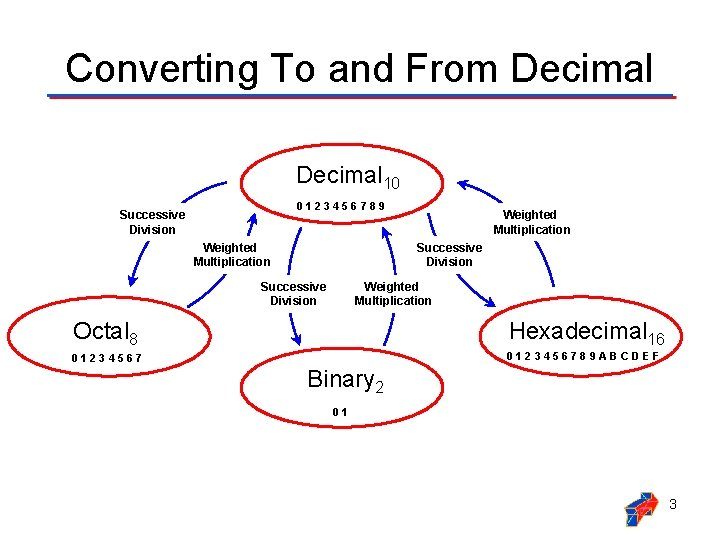Converting To and From Decimal 10 0123456789 Successive Division Weighted Multiplication Octal 8 Hexadecimal 16 0123456789 ABCDEF Binary 2 01 3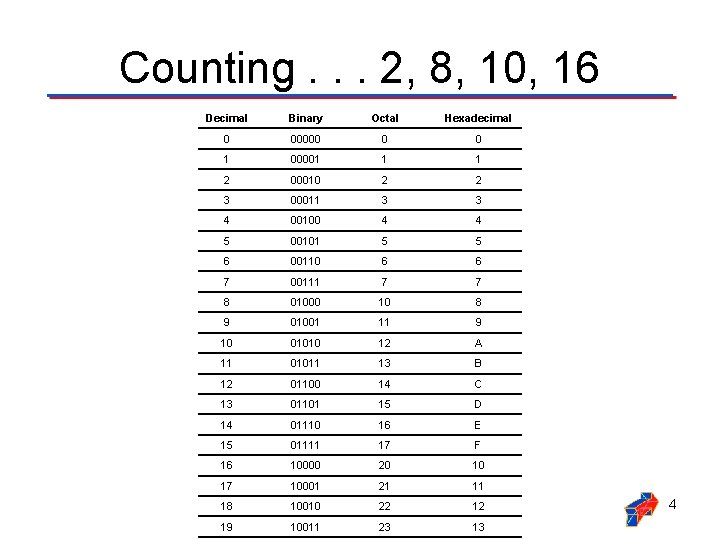Counting. . . 2, 8, 10, 16 Decimal Binary Octal Hexadecimal 0 00000 0 0 1 00001 1 1 2 00010 2 2 3 00011 3 3 4 00100 4 4 5 00101 5 5 6 00110 6 6 7 00111 7 7 8 01000 10 8 9 01001 11 9 10 01010 12 A 11 01011 13 B 12 01100 14 C 13 01101 15 D 14 01110 16 E 15 01111 17 F 16 10000 20 10 17 10001 21 11 18 10010 22 12 19 10011 23 13 4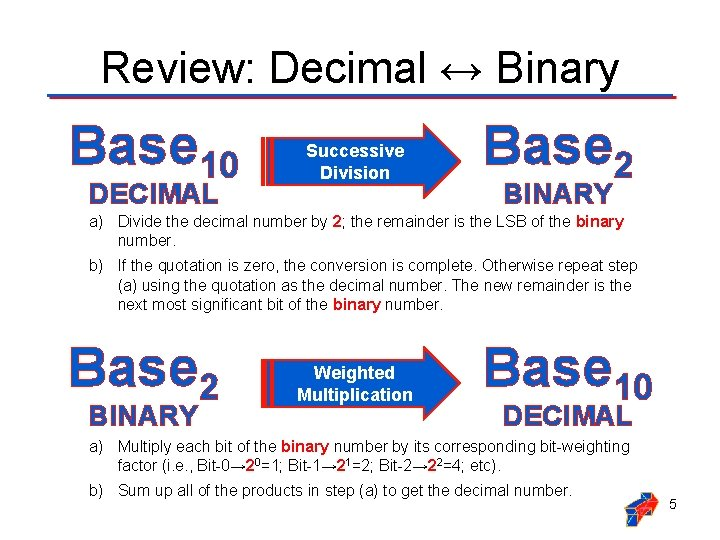Review: Decimal ↔ Binary Base 10 DECIMAL Successive Division Base 2 BINARY a) Divide the decimal number by 2; the remainder is the LSB of the binary number. b) If the quotation is zero, the conversion is complete. Otherwise repeat step (a) using the quotation as the decimal number. The new remainder is the next most significant bit of the binary number. Base 2 BINARY Weighted Multiplication Base 10 DECIMAL a) Multiply each bit of the binary number by its corresponding bit-weighting factor (i. e. , Bit-0→ 20=1; Bit-1→ 21=2; Bit-2→ 22=4; etc). b) Sum up all of the products in step (a) to get the decimal number. 5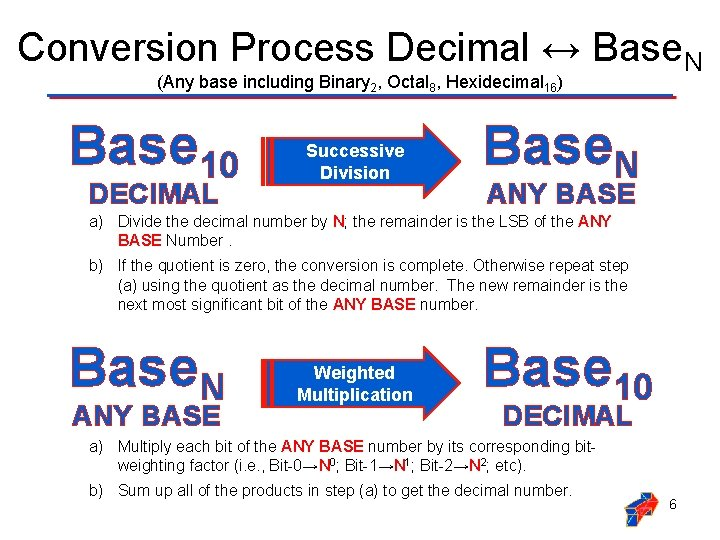Conversion Process Decimal ↔ Base. N (Any base including Binary 2, Octal 8, Hexidecimal 16) Base 10 DECIMAL Successive Division Base. N ANY BASE a) Divide the decimal number by N; the remainder is the LSB of the ANY BASE Number. b) If the quotient is zero, the conversion is complete. Otherwise repeat step (a) using the quotient as the decimal number. The new remainder is the next most significant bit of the ANY BASE number. Base. N ANY BASE Weighted Multiplication Base 10 DECIMAL a) Multiply each bit of the ANY BASE number by its corresponding bitweighting factor (i. e. , Bit-0→N 0; Bit-1→N 1; Bit-2→N 2; etc). b) Sum up all of the products in step (a) to get the decimal number. 6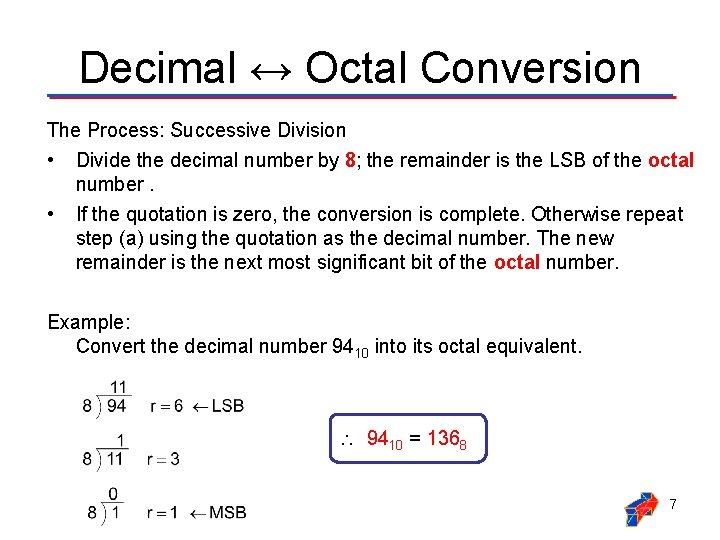Decimal ↔ Octal Conversion The Process: Successive Division • Divide the decimal number by 8; the remainder is the LSB of the octal number. • If the quotation is zero, the conversion is complete. Otherwise repeat step (a) using the quotation as the decimal number. The new remainder is the next most significant bit of the octal number. Example: Convert the decimal number 9410 into its octal equivalent. 9410 = 1368 7Example: Dec → Octal Example: Convert the decimal number 18910 into its octal equivalent. 8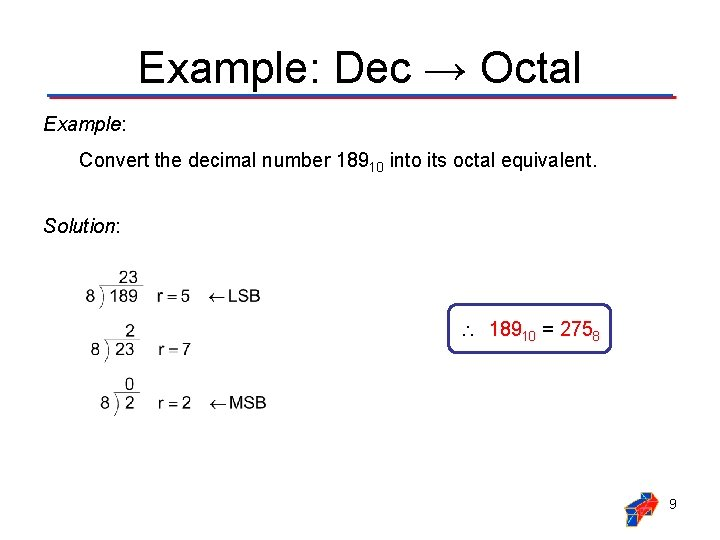Example: Dec → Octal Example: Convert the decimal number 18910 into its octal equivalent. Solution: 18910 = 2758 9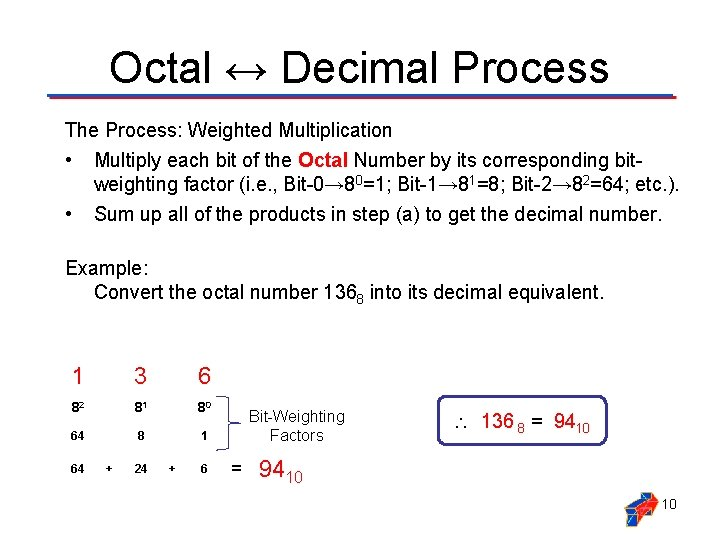Octal ↔ Decimal Process The Process: Weighted Multiplication • Multiply each bit of the Octal Number by its corresponding bitweighting factor (i. e. , Bit-0→ 80=1; Bit-1→ 81=8; Bit-2→ 82=64; etc. ). • Sum up all of the products in step (a) to get the decimal number. Example: Convert the octal number 1368 into its decimal equivalent. 1 3 6 82 81 80 64 8 1 64 + 24 + 6 Bit-Weighting Factors = 136 8 = 9410 10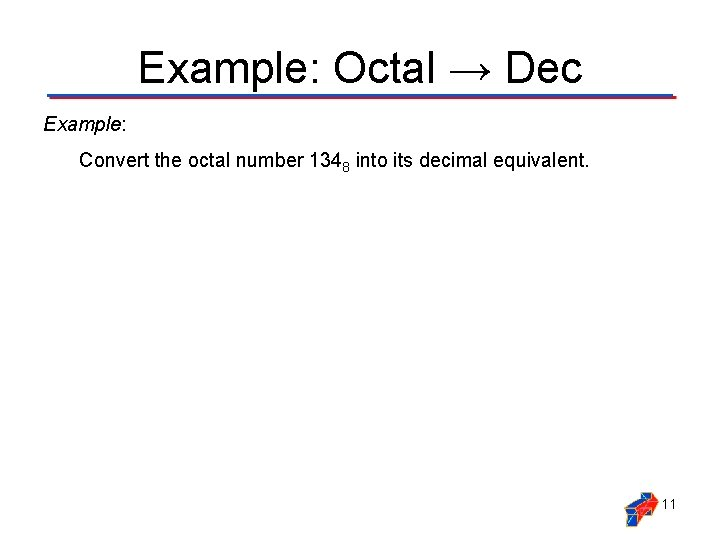Example: Octal → Dec Example: Convert the octal number 1348 into its decimal equivalent. 11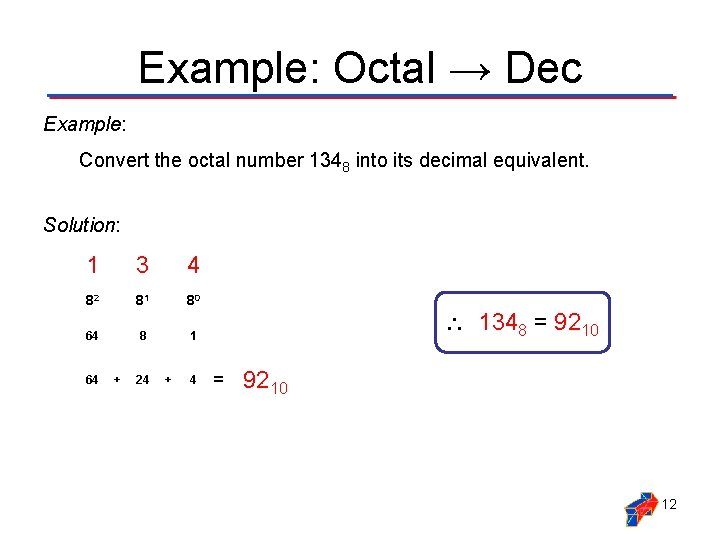Example: Octal → Dec Example: Convert the octal number 1348 into its decimal equivalent. Solution: 1 3 4 82 81 80 64 8 1 64 + 24 + 4 1348 = 9210 12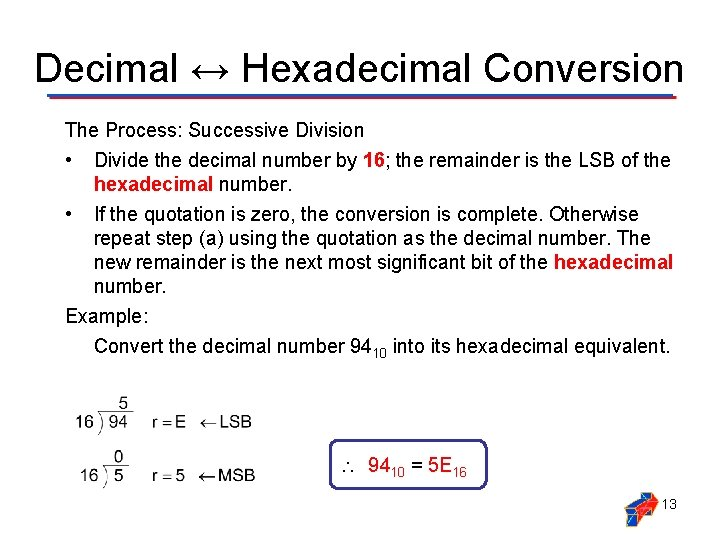Decimal ↔ Hexadecimal Conversion The Process: Successive Division • Divide the decimal number by 16; the remainder is the LSB of the hexadecimal number. • If the quotation is zero, the conversion is complete. Otherwise repeat step (a) using the quotation as the decimal number. The new remainder is the next most significant bit of the hexadecimal number. Example: Convert the decimal number 9410 into its hexadecimal equivalent. 9410 = 5 E 16 13Example: Dec → Hex Example: Convert the decimal number 42910 into its hexadecimal equivalent. 14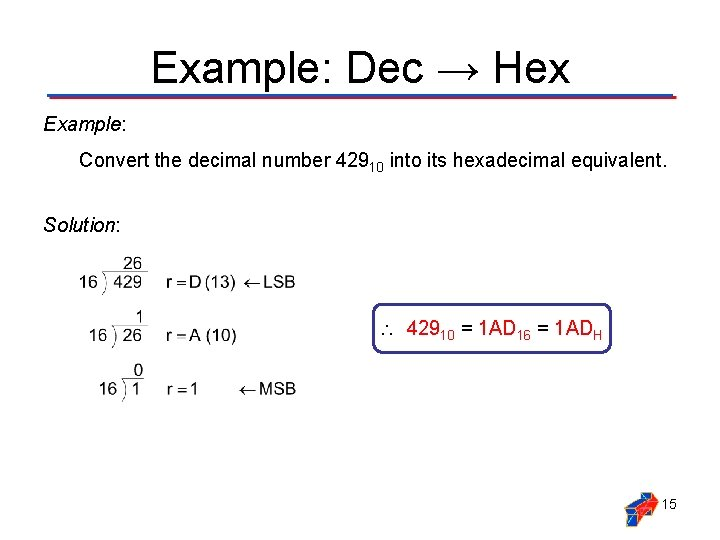Example: Dec → Hex Example: Convert the decimal number 42910 into its hexadecimal equivalent. Solution: 42910 = 1 AD 16 = 1 ADH 15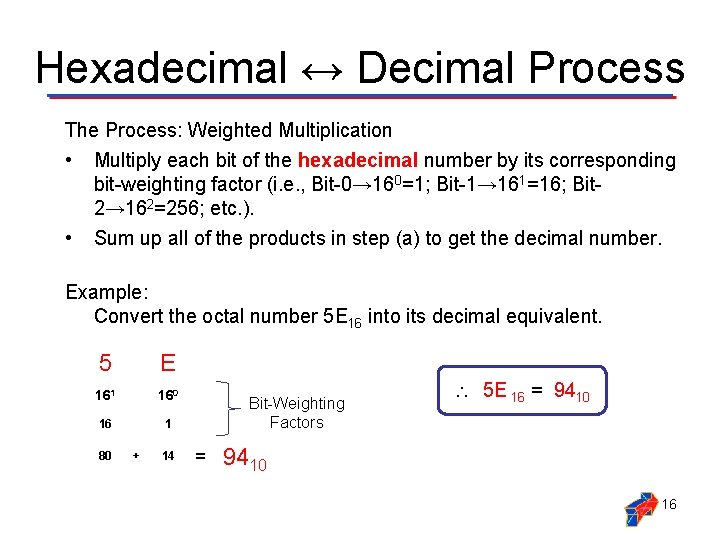Hexadecimal ↔ Decimal Process The Process: Weighted Multiplication • Multiply each bit of the hexadecimal number by its corresponding bit-weighting factor (i. e. , Bit-0→ 160=1; Bit-1→ 161=16; Bit 2→ 162=256; etc. ). • Sum up all of the products in step (a) to get the decimal number. Example: Convert the octal number 5 E 16 into its decimal equivalent. 5 E 161 160 16 1 80 + 14 Bit-Weighting Factors = 5 E 16 = 9410 16Example: Hex → Dec Example: Convert the hexadecimal number B 2 EH into its decimal equivalent. 17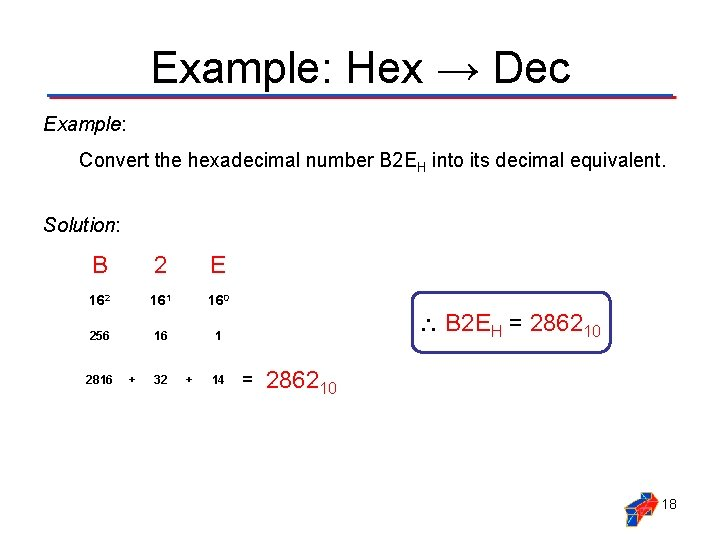Example: Hex → Dec Example: Convert the hexadecimal number B 2 EH into its decimal equivalent. Solution: B 2 E 162 161 160 256 16 1 2816 + 32 + 14 B 2 EH = 286210 18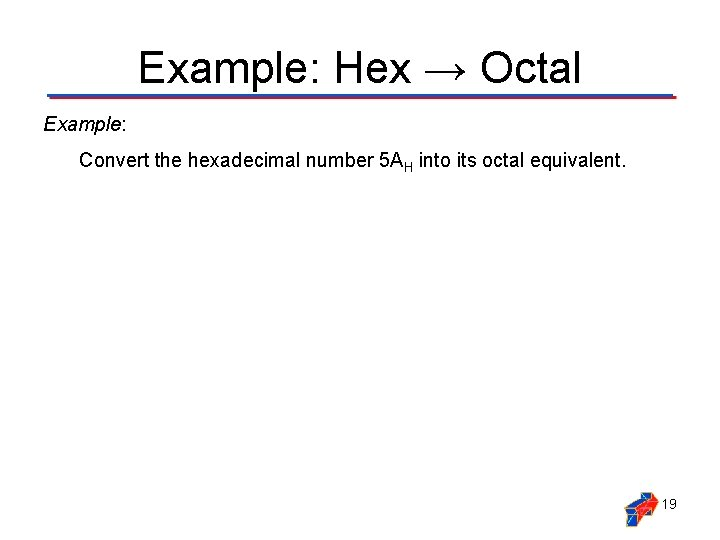Example: Hex → Octal Example: Convert the hexadecimal number 5 AH into its octal equivalent. 19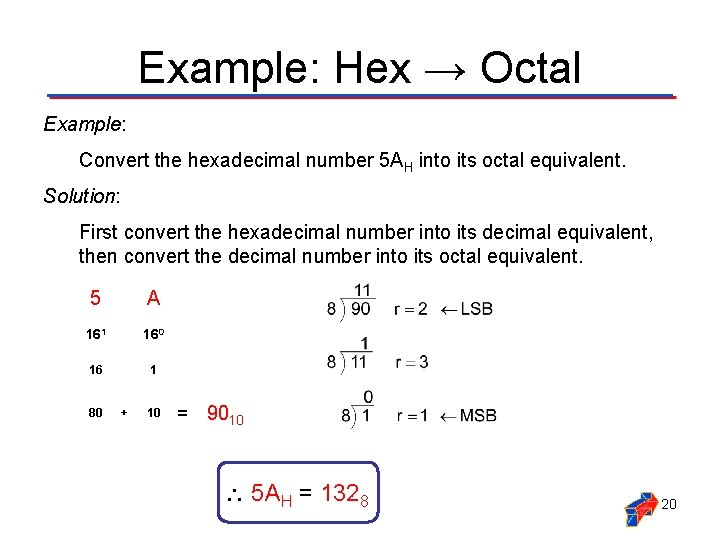Example: Hex → Octal Example: Convert the hexadecimal number 5 AH into its octal equivalent. Solution: First convert the hexadecimal number into its decimal equivalent, then convert the decimal number into its octal equivalent. 5 A 161 160 16 1 80 + 10 = 9010 5 AH = 1328 20Example: Octal → Binary Example: Convert the octal number 1328 into its binary equivalent. 21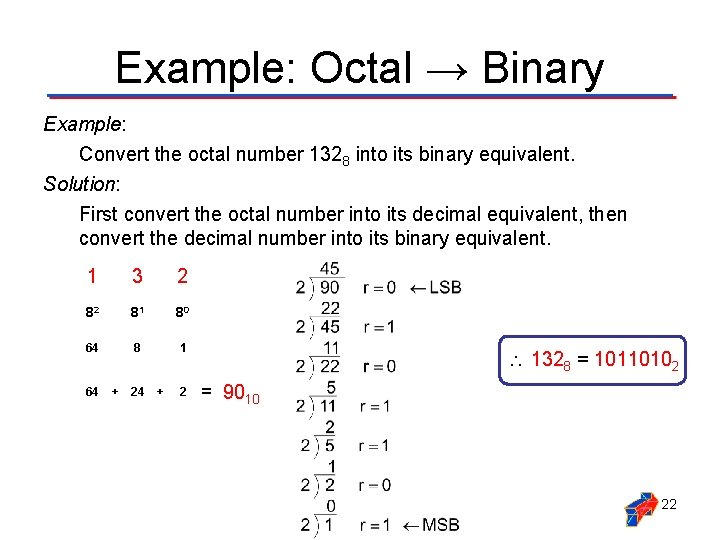Example: Octal → Binary Example: Convert the octal number 1328 into its binary equivalent. Solution: First convert the octal number into its decimal equivalent, then convert the decimal number into its binary equivalent. 1 3 2 82 81 80 64 8 1 64 + 2 1328 = 10110102 = 9010 22Binary ↔ Octal ↔ Hex Shortcut Because binary, octal, and hex number systems are all powers of two (which is the reason we use them) there is a relationship that we can exploit to make conversion easier. 1 0 1 0 2 = 132 8 = 5 A H To convert directly between binary and octal, group the binary bits into sets of 3 (because 23 = 8). You may need to pad with leading zeros. 0 0 1 1 0 2= 1 3 28 1 3 2 001 010 To convert directly between binary and hexadecimal number systems, group the binary bits into sets of 4 (because 24 = 16). You may need to pad with leading zeros. 0 1 1 0 2= 5 5 A A 16 0101 1 010 23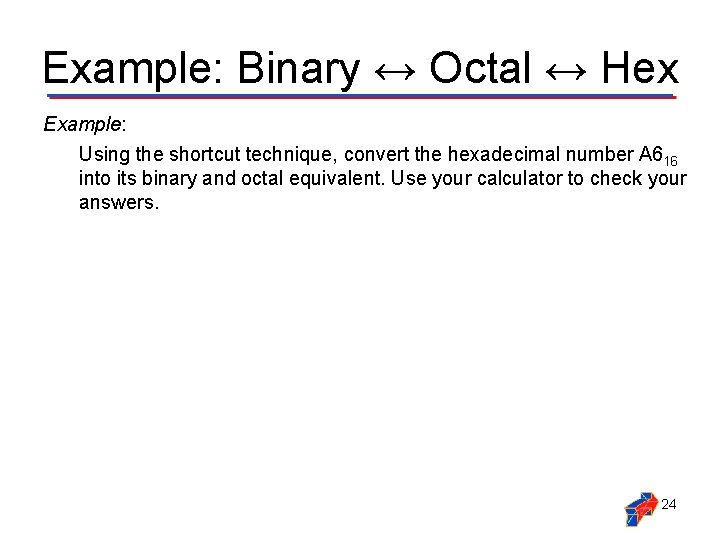Example: Binary ↔ Octal ↔ Hex Example: Using the shortcut technique, convert the hexadecimal number A 616 into its binary and octal equivalent. Use your calculator to check your answers. 24Example: Binary ↔ Octal ↔ Hex Example: Using the shortcut technique, convert the hexadecimal number A 616 into its binary & octal equivalent. Use your calculator to check your answers. Solution: First convert the hexadecimal number into binary by expanding the hexadecimal digits into binary groups of (4). A 6 16 1010 0110 A 616 = 101001102 Convert the binary number into octal by grouping the binary bits into groups of (3). 010100110 2 4 6 101001102 = 2468 25